﻿ Using Artificial Neural Network to Model Water Discharge and Chemistry in a River Impacted by Acid Mine DrainagePublications are Open
Access in this journal
Article Versions
Export Article
• Normal Style
• MLA Style
• APA Style
• Chicago Style
Review Article
Open Access Peer-reviewed

### Using Artificial Neural Network to Model Water Discharge and Chemistry in a River Impacted by Acid Mine Drainage

Toluwaleke Ajayi , Dina L.Lopez, Abiodun E.Ayo-Bali
American Journal of Water Resources. 2021, 9(2), 63-79. DOI: 10.12691/ajwr-9-2-4
Received August 28, 2021; Revised October 02, 2021; Accepted October 11, 2021

### Abstract

In southeast Ohio, Raccoon Creek Watershed (RC) has an extensive mining history resulting in acid mine drainage (AMD) and subsequent environmental problems. Modeling of the discharge and chemistry of AMD impacted Hewett Fork, a tributary of Raccoon Creek, is the focus of this paper. Discharge measurements are collected by the United States Geological Survey (USGS) gage station at the Bolin Mills (BM) station on the main stem of RC. This data for the period 2011-2019 has been analyzed to develop a prediction model for BM discharge and subsequently use the model to predict flow and water chemistry in Hewett Fork (HF). The Neural Network Model using group method of data handling (GMDH) and generalized regression neural network (GRNN) shows a variation of prediction models for BM due to parameters such as decay factor in API and ATI, as well as in the evapotranspiration input variables of the model. However, the study reveals that the GRNN model for BM is the most suitable for BM prediction based on its performance, with an r-value greater than 0.90, and its ease in predicting discharge by specifying a data set to be added to the data set for training and calibrating the network. The result of the water chemistry model using GMDH, with r values greater than 0.80 for each model, shows the input variables have a good capacity to predict chemical concentration/load in the HF stream. This study shows that Artificial Neural Network (ANN) modeling can help to model successfully and predict flow and chemical evolution of rivers in Ohio and other parts of the world.

### 1. Introduction

For over 200 years, coal mining has been a major economic source in the eastern United States. As a result of the rise in a coal mining operation in the eastern region which includes Ohio, acid mine drainage (AMD) became rampant due to the high sulfide content of coal and associated rocks thereby posing severe environmental pollution problems for the coal mining communities 1. AMD is a major and widespread environmental pollution problem in the Appalachian region. Pennsylvania, Ohio, and West Virginia are the states within the Appalachian region that are characterized by having series of multiple coal layers along their geologic strata 2. The oxidation of sulfide mineral ores is the main source of AMD, which is initially exposed to the surrounding environment because of intensive mining activities. AMD has a low pH, high total dissolved solids (TDS), is net acidic, and normally has elevated concentrations of manganese, sulfate, aluminum, and iron 3. The impact of AMD in nearby streams is that the stream water quality is degraded, and the stream's biological activity is negatively affected.

An AMD impacted river found in southeast Ohio is the Raccoon Creek watershed, with approximately 9-10 percent of the total watershed mined 4. A matter of concern in this impacted stream is the movement of contaminants and sediments because the precipitation of metallic compounds acidifies the stream and also increases the sediment load. In order to have a better understanding of the movement of contaminants, nutrients, and sediments in the stream, it is important to understand the flow regime, chemical transport, and also generate streamflow predictions. The prediction of streamflow is expedient for the planning and management of water resources. In a situation where a river basin has a scarce record of hydrologic data, which could be as a result of them not being gaged or partially gaged, water resource engineers and hydrologist depends solely on the modeling of past empirical data to make streamflow predictions. Generally, such predictions are useful in remediation studies, flood, or drought prediction, and for the optimization of hydrologic systems of a basin.

Most analysis of hydrological systems involves the mapping and modeling of non-linear data, which is performed by statistical tools such as curve fitting and regression. However, in a situation where the underlying physical law is not well known or it is too complex, it becomes difficult to effectively model the phenomenon 5. Effort has been made in applying computational techniques that do not require rule development, thereby reducing software complexity; one of such computational technique is called neurocomputing, and the network that performs this neurocomputation is called Artificial Neural Networks (ANN) 5. A major advantage that ANN has over other traditional methods is that the complex nature of the underlying process is not required to be described explicitly in a mathematical format 6. ANN is therefore described as a universal approximator that can successfully learn from examples or past experience without explicit physics 7. ANN models are easily developed and can generate satisfactory results especially when they are applied to a complex system that is not well understood or poorly defined 8.

ANN has been applied in hydrology for forecasting in hydraulics and environmental prediction 9 water quality assessment 10 forecasting streamflow 11, 12, modeling reservoir operations 13, rainfall-runoff modeling 14. In a broader context, in one of the early applications of ANN to streamflow predictions, Kang et al. 15 used a combination of autoregressive moving average model (AMAM) and ANN using different three-layer design architecture for the prediction of hourly and daily streamflows in Pyung Chang River basin in Korea. The authors concluded that ANNs help predicts streamflows. Another study relating to streamflow prediction was conducted by Karunanithi et al. 16 at an ungaged site in the Huron River, Michigan, with data based on a USGS stream gage station located downstream of the sampling site. These authors mentioned that ANN has the adaptive capability to accommodate historical changes in streamflow records. In general, all ANN models can produce a better result compared to other types of models like linear regression and are better than deterministic models.

In this paper, the discharge and chemistry of Hewett Fork (HF), a stream impacted by AMD in Raccoon Creek, is investigated based on data collected during the last 9 years. The objective of this study is to use the ANN model to understand and highlight important hydrologic parameters that can influence and predict discharge in a gaged flow (USGS Bolin Mills) and subsequently used those parameters to calibrate flow, and water chemistry in the ungaged stream (HF flow). Modeling of flow and water chemistry in an AMD-affected stream is needed to understand how the effects of change in hydrologic parameters affect water quality. It is a major step to simulate other processes happening in the river and to also have a better understanding of the physical environment, biological recovery, and water chemistry of the stream. The modeling studies are also important in understanding stream recovery and making a better future remediation plan. For instance, change in stream discharge from modeling extreme events can help to know if over-treating or undertreating is happening in the treatment process of the streams and how all these changes in the treatment process affect the quality of stream during extreme events.

### 2. Artificial Neural Network

ANN is a computational system developed by individual cells that carry out computational calculations which is similar to how the human brain functions 17. It can learn patterns and also use this information to provide solutions to problems with nonlinearity and high-level complexity 18. In a typical ANN, each node is arranged in a particular order. In a feedforward network, the arrangement of nodes generally starts from the first input layer and ends at the final output layer 18. There is also the possibility of having several hidden layers in the network, with each layer containing one or more nodes 19. Information is passed from the input side to the output side. The nodes in a layer are not connected to those in the same layer but are only connected to nodes in the next layer. Thus, the inputs received from the previous layer and the corresponding weight determines the output of a node in a layer 20. In most neural network models, the first input variable is received in the first input layer, with each input variable containing all quantities that can directly or indirectly influence the output 21. As a result of this, the input layer is transparent because it provides all the necessary information that the network needs 21. The output layer which consists of predicted values by the network is referred to as model output. The network uses a trial-and-error procedure to determine the overall hidden layers and the number of nodes each hidden layer contains 19. The nodes within each neighboring layer of the network are completely attached by connected links. A synaptic weight is allocated to each connection link, representing the relative strength of the connection of two nodes at both ends to predict the relationship between the input-output 19. Figure 1 shows the configuration and topology of a three-layer ANN. These kinds of feedforward three-layer ANN have been applied to a variety of problems, such as pattern classification, recalling and storing data, solving constrained optimization problems, and grouping similar patterns 22. In this Figure, X represents the input vector that consists of several variables that influence the behavior of the system while Y represents the output vector that consists of the resulting variable that describes the behavior of the system.

• Figure 1. Configuration and topology of ANN 

Figure 2 shows a schematic diagram of a typical jth node. Depending on the location of a node in a layer, the node’s input structure is generated from casual system variables or the outputs of other nodes 21. These input systems then generate an input vector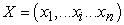21. The weight sequence leading to the node generates a weight vector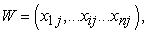where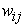represents the weight of the connection from the jth node 21.

• Figure 2. Schematic diagram of a typical jth node 

The node’s output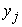can be obtained when the function f values are computed for vector X and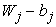where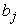is called the bias (also known as threshold value) that is connected to this node 22. The operation explained above is defined in equation 1.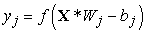(1)

For an output vector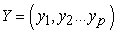generated by ANN to be close to the target vector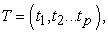a learning process, also called training, is applied to the network to find the bias vector V and weight matrices W, minimizing error function in the form expressed in equation 2 22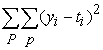Where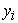is the corresponding output of ANN,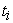represents the components of output T (the observed values), P is the number of patterns trained and p is the number of node’s output 22. Network training is defined as the process by which ANN connection weights are adapted through a continuous simulation process by the environment in which the network is set 23. The effect variables of the system are the output while the cause variable is the inputs. The procedure of the training involves optimization and iterative adjustment of threshold values and connection weight for each node 22. After the network has been successfully trained, it is hoped that the network produces a good and reasonable result.

In this paper, the ANN model was conducted using a commercially available simulation package, NeuroShell®2 24. NeuroShell®2 uses the training data to “learn” patterns, allowing it to make predictions when new data is presented. The ANN network design architecture applied in this study is Group Method of Data Handling (GMDH) 25 and Generalized Regression Neural Network (GRNN) 26 in the NeuroShell®2 program.

GMDH produces a mathematical formula that is expressed as a non-linear polynomial, which relates the values of the most significant input variables used in predicting the output variable 25. It uses a link of a simple polynomial to build a successive layer. It creates this layer by computing regressions of the input variables and then proceeds to select the best ones called survivors 25. GMDH is a powerful design module that creates a great deal of flexibility in configuring variables that are used to train the network. However, the complexity of the polynomial, which can include dozens of terms, often makes the equation difficult to apply.

GRNN is more structurally significant when compared to other neural network design architecture 27. It learns very fast using a simple design technique to produce a good regression point 26. It does not make an ambiguous model prediction. Furthermore, due to the design's toughness and simplicity, it can make a quick calibration and verification. With GRNN, you do not get a polynomial equation that you can apply later to another data set. You have to run the model and add the prediction input data to obtain results for the prediction of the dependent variable.

### 3. Description of Model Variables

The interaction between the hydrological processes involves several meteorological variables such as air temperature, precipitation, solar radiation, and evapotranspiration 28. For this modeling study, it is important to understand how precipitation, air temperature, Antecedent Precipitation Index (API), and evapotranspiration affect discharge. With most precipitation falling as rain, it is a major connection in the water cycles that determines how atmospheric water is delivered to the earth 29. The climatic effect on river discharge is due to temperature and precipitation. Generally, precipitation rates are significant factors affecting discharge quantity 30. However, the ways such precipitation occurs plays a major role in the quantity of this discharge 30. For instance, evapotranspiration and infiltration tend to be decreased when heavy (intensive and frequent) rain occurs, causing a large amount of water to flow into the stream (runoff) 30. Stream discharge is affected by this type of precipitation. The discharge decreases if precipitation occurs in the form of snowfalls 31. In the USA, discharge often occurs during the early winter to late spring. The reason is that around the period of November to April, most precipitation occurs, and evapotranspiration is low, falling like rain into the drainage system 32. Furthermore, the decrease in discharge during the summer months may not only be attributed to a low precipitation rate but may be due to a high rate of evaporation and active growth of shoreline vegetation, thereby removing water from the ground 33.

Temperature is another factor that may affect discharge. It plays a significant role in snowmelt and evapotranspiration. Depending on the season of the year, an increase in air temperature will greatly affect discharge 34. High temperature during the summer tends to increase evaporation rates, leading to a reduction of river discharge 34. In the winter season, the warmer period may give rise to a faster rate of snowmelt when there is snowpack, or it may cause precipitation to take place in the form of rain rather than snow 35. As a result, the discharge could increase during winter, especially at the end of the season.

When there are no stream gages in watersheds, then it becomes difficult to provide accurate records of daily discharge. In such an ungaged watershed, daily discharge can be predicted using rainfall-runoff models and statistical tools. In watershed management, rainfall-runoff modeling is an important tool in streamflow prediction. Prediction of peak flows in a watershed is useful in restoring channel habitat structures. The use of the Antecedent Precipitation Index (API) as a key variable for predicting discharge has been proven effective in environments with no sufficient data 36. API was originally conceived to show current soil moisture in storm volume prediction models 36. It works on the theory that earlier precipitation will have a low influence on current streamflow response as compared to recent precipitation 36. The decay factor is used to represent the “memory effect” of a watershed by decaying the impact of accumulated rainfall for each time. A short-term API demonstrates a more recent rainfall intensity that governs response to peak flow while a long-term API demonstrates seasonal moisture condition 37.

Evapotranspiration, as a major process in the climatic system, links the energy budget, carbon cycle, and hydrological cycle together 38. Factors that may affect the rate of evapotranspiration include wind, solar radiation, soil moisture, and temperature. With temperature being one of the most significant variables affecting discharge, the rate of transpiration goes up as air temperature increases 39. This increased temperature may cause the stomata in the plant cell to open, thereby releasing water to the atmosphere whereas the opening closes when the temperature is colder 40.

In this paper, the model variables applied to predict discharge and stream chemistry are temperature °C, precipitation (inch), API (inch), ATI (°C), and evapotranspiration (mm/day).

### 4. Site Description and Geologic Setting

4.1. Site Description

The Raccoon Creek watershed (RC) covers about 1770 square kilometer and is also about 180 km long 41. RC is approximately 75.5% forested and with a gradient of 3.2ft per mile. Before the Surface Mine Control and Reclamation Act in 1977, both underground and surface coal mine impacts were neglected within the watershed 41. In recent years, several organizations have tried to remediate the AMD pollution produced from these mines, the Raccoon Creek partnership in conjunction with many partnering organizations has been working on remediating AMD and restoring the watershed to its pre-mining state 42. HF watershed (a sub-watershed of RC) is located in Ohio within Athens and Vinton County (Figure 3a). The reason why we selected this stream for this modeling is because it is the most studied stream in SE Ohio. Discharge and chemistry have been investigated frequently in this stream but not in a continuous long-term manner. However, there is a USGS station in the main stem of Raccoon Creek, the Bolin Mills (BM) gage station. The discharge measurement of the USGS BM gage station is of interest for this paper because it can be used to determine the discharge at HF if the drainage areas of the different sampling stations at HF are known. HF is the fourth largest tributary to RC 41. HF’s main stem has a length of about 15.4 miles and has a drainage area of 40.51 square miles 41. RC has a stream length of about 108 miles. It drains an approximate area of 683.5 square miles 41. The topography of RC is made up of a narrow ravine, narrow stream valleys, attenuated ridges, and steep hillsides which tend to constrain the stream floodplains 43.

• Figure 3. a: Map of RC watershed, showing HF subwatershed. The blue circle is the BM gage station and black circle is location of doser ; b: HF sampling points and biologic recovery zones 

The major source of AMD that enters the HF watershed is from two deep mine portals of mine complex AS-14 in Carbondale, Ohio 41. In the 1980s, Carbondale mine seeps were made a priority for treatment by the Ohio Department of Natural Resources Division of Mineral Resource Management 4. As Carbondale Seeps remained a major remediation priority, an Aquifix lime-doser was installed in 2004 and the purpose of the doser is to add calcium oxide to the stream to neutralize the AMD. After the installation of the doser, studies conducted by DeRose 44 and Kruse et al. 45, reveal that HF stream had significant improvements in pH, alkalinity, fish, and macroinvertebrate communities. On this note, the HF watershed is divided into three biologic recovery zones based on aquatic health (Figure 3b), and they are the impaired zone, transition zone, and improved zone 41. The impaired zone is characterized to have poor biological quality because of the severe impact of AMD and the addition of heavy alkaline from the doser. The fishes and macroinvertebrates began showing improvements in the transition zone while the improved zone, which is at the confluence of HF and RC, can sustain biological life 41.

4.2. Geologic Setting

HF subwatershed lies in the unglaciated portion of Ohio. The bedrock of the watershed is made up of sedimentary rock from the Pennsylvanian and Mississippian periods 46. The Mississippian is made up of the Logan and Cuyahoga rock members which include shale, conglomerate, and sandstone 47. The Allegheny Formation includes coal, sandstone, shale, and some limestone formed during the Pennsylvanian 47. Throughout the state of Ohio, the Allegheny Formation is a prime coal resource that consists of three important thick coal beds that are rich in sulphides 48. Lower Kittanning No. 5, the Clarion No. 4a, the Middle Kittanning No. 6 are the coal seams of interest in southeast Ohio, and they are the coal seams that are mineable in Hewett Fork 48. The topography of the RC watershed is made up of a narrow ravine, narrow stream valleys, attenuated ridges, and steep hillsides which tend to constrain the stream floodplains 43. Soil erosion occurs in the watershed because of the steep hillsides which increase the stream sedimentation, thereby increasing the stream sediment load in addition to any AMD pollution in the stream. Clay-rich soils are the type of Soil in southeast Ohio. The impact of Clay type soils is significant on the infiltration capacity, lower and upper zone nominal soil storage. When these clay particles become full of water, they tend to swell and act as an impermeable layer 49. Land types also affect hydrology calibration. Forested land has a lot of evapotranspiration and interception during the growing season while agricultural land uses have varying evapotranspiration, monthly values for interflow, interception, and infiltration. Depending on the time of the year, agricultural land use can have a changing infiltration parameter. The parameter for non-vegetated land use remains constant all year due to little or no change in the characteristics of the land use 50. The watershed area to the BM gage point consists primarily of forest and agriculture.

### 5. Methodology

ANN modeling enabled us to determine the impact of variations in parameters (e.g., API, precipitation, air temperature, and evapotranspiration) and how changes to these parameters affect the modeling of flow in BM and HF and chemical transport in HF.

5.1. Data Gathering for Flow Model in BM

Discharge measurements collected by the USGS gage station at BM station were extracted from the USGS National Water Information system (NWIS) www.waterdata.usgs.gov/nwis. Precipitation and daily air temperature data for the 9 years study period were retrieved from Scalia Laboratory for Atmospheric Analysis (SLAA) at Ohio University. Antecedent precipitation index (API) for the study period was calculated using the equation 3 and 4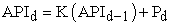(3)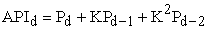(4)

Where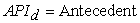Precipitation index for any day, K = Decay factor (< 1) and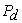= Precipitation for day d.

In order to include a longer impact of temperature in the river discharge, a new concept similar to API is introduced in this paper and it is called antecedent temperature index (ATI). The ATI used in this study was calculated using Equations 5 and 6.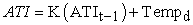(5)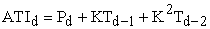(6)

Where ATI is the antecedent temperature index of the day,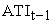is the antecedent temperature of the previous day and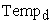is the temperature of the day.

Potential evapotranspiration was calculated using both Blaney-Criddle Method and Hargreaves Method. The Blaney-Criddle 51 process of estimating potential evapotranspiration (ET) has been used extensively especially in the western USA 52. The Blaney-Criddle equation used for the calculation is expressed in equation 7: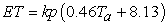(7)

Where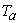= Daily mean temperature in °C, ET=potential evapotranspiration (mm/day), p= percentage of the total daytime hour, and k=monthly consumptive coefficient.

ET calculated using Hargreaves 53 equation is expressed in equation 8: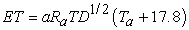(8)

Where TD = difference in the maximum and minimum daily temperature (°C), a = 0.0023 and Ra= extraterrestrial radiation in mm/day.

5.2. Data Gathering for Flow and Chemical Model for HF

Discharge data between 2011-2019 for HF sampling points HF010, HF039, HF060, HF090, HF137, HF190 was extracted from www.watersheddata.com and previous work in HF from Mckay 54. This web application, which is a product of Ohio University Voinovich School of Leadership and Public Affairs, is an important source of water quality data in Ohio. The chemical data available in Hewett fork includes pH lab, acidity, ORP, alkalinity, TDS, sulfate, total Ca, total Na, total K, total Fe, total Mn, total Al, total Cl, and hardness for sampling sites HF010, HF039, HF060, HF090, HF095, HF131, HF137, and HF190. See Figure 3b for the location of sampling points in HF. All the data for this chemical parameter for the 9-year study period at each sample site were also extracted from www.watersheddata.com. However, it should be noted that the chemical and discharge data for HF are not continuous; the parameters have been determined and reported only a few times during the year while in BM, there is continuous flow measurements at small time intervals but reported every hour by USGS.

5.3. ANN Model Development for Flow in BM

ANN was used in this study to show how some hydrological parameters influence discharge in BM. BM flow was modeled in three approaches. The first approach involves modeling using GMDH, with BM discharge as the output variable while the input variables are precipitation, API, temperature, separated values of temperature, and potential evapotranspiration (ET). For flow in BM, a training pattern is defined. This represents the total data inputs into the network, with each set containing data for the input and output variable for the study period. NeuroShell®2 then calibrates these variables using GMDH. The main concept behind GMDH is that it builds a polynomial model function that would behave in a way that the predicted value would be close as much as possible to the actual value, making it easier for users to be able to make a better prediction using a polynomial formula that the user is familiar with.

In the second approach, the Antecedent Temperature Index (ATI) is introduced. The use of ATI is a more simplified and logical way of expressing previous temperature values as one input variable, as opposed to separated values of temperature where more than one input variable is used, depending on the number of days considered. In this approach, GMDH is the network design used for the model, with BM discharge as the output variable while ATI, API, precipitation, temperature, and ET are computed as input variables. A similar procedure for the GMDH model used in the first approach is also employed in the second approach.

In the third approach, the ANN model was carried out using GRNN. In GRNN, the program extracts a test set and production set from the training pattern. GRNN was selected because it could provide a lower error than GMDH. The test set helps to prevent the network from overtraining the data so that it can generalize well on a new set of data. The network uses a production set to test the result of the network model with data that the network has not processed before. A summary of the model arrangement for flow in BM is presented in Table 1.

5.4. ANN Development for Flow in HF

Hydrologic modeling can be used to predict discharge in ungaged streams. In this scenario, a hydrologically similar gaged stream close to the ungaged stream is used to calibrate the model. The model parameters are adjusted in a way that reflects the physical changes between the ungaged watershed and calibration watershed. In this paper, discharge measurements collected by the USGS gage station at BM station are used to calibrate the flow in the HF stream, which is near-by to the BM station. Discharge data for HF010, HF039, HF060, HF090, HF137, HF190, and their river mile and drainage area were the parameters extracted for the HF stream. For dates where discharge data are available for each HF site between 2011-2019, BM discharge, temperature, ATI, API, and precipitation were also extracted for those dates since there is continuous data for them. ANN using GMDH was used for the modeling, with HF discharge as output variables while BM discharge, temperature, ATI, API, river mile, drainage area, and precipitation are input variables. In the end, a long streamflow record can be created from the polynomial equation generated by the network. The polynomial equation represents the formula that can help predict the discharge at HF stream (an ungaged AMD stream), provided all other input parameters are available.

5.5. ANN Development for HF Chemical Model

The first step was to extract chemical data such as acidity, aluminum (Al), potassium (K), iron (Fe), alkalinity, magnesium (Mg), calcium (Ca), and manganese (Mn), available for each HF site. The extraction is divided into two stages. The first stage is to extract chemical data for dates where discharge data is available in HF010, HF039, HF090, and HF190. The second stage is to extract chemical data for dates where chemical data is collected but the discharge was not measured. This stage involves introducing new HF sites such as HF060, HF095, and HF137, where discharge data was not measured but has abundant chemical data. The polynomial equation (formula) generated for the flow in the HF stream is then used to predict the discharge (simulated discharge) for dates where there is abundant chemical data, but discharge data is not available. The mathematical formula was used to predict discharge in 36 additional dates where chemical data is abundant, but discharge is not measured, which gives a total of 96 data points (both measured and simulated discharge) that was considered in this study. The third stage is to gather all data in the first and second stages together i.e all chemical data for each site, HF discharge (both measured and simulated discharge), precipitation, API, BM discharge, temperature, ATI, drainage area, and river mile. ANN using GMDH was then applied, with each chemical parameter (chemical concentration and load) as output variable while BM discharge, precipitation, API, temp, ATI, river mile, and drainage area were computed as input variables. The chemical load in kg/day was calculated using the expression in equation 9: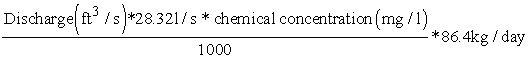(9)

Upon completion of the ANN model run, a graph of %error for each chemical concentration and load vs discharge was plotted. As a result of the findings from this error plot, the model was divided into two categories based on HF discharge. The % model error is calculated using the expression in equation 10.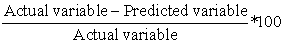(10)
5.6. Model Performance Evaluation

Models were evaluated using R squared (Coefficient of determination), Root means square error (RMSE), and correlation coefficient (r-value), which was generated in the Neuroshell2 program. Furthermore, after the training and testing of the neural network models have been completed, the model with the best performance was validated by comparing the actual value to the predicted value by the network.

### 6. Results and Discussion

6.1. Modeling of Flow in BM

In the first approach to modeling BM discharge, BM discharge was computed as output variable while API with a decay factor of 0.95, precipitation, temperature,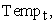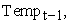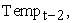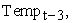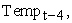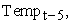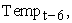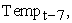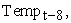and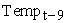was computed as input variables (model BM 1). Studies conducted by Raju et al. 19 have also used separated values of temperature as predictor variables for the simulation of spring discharge and their result show a good model output. Initially, a constant year-round decay constant (k) of 0.95 was used in the API calculation for this paper based on the recommendation of Hill et al. 55. Lindsay et al. 56 stated that decay constant (k) must not be greater than one. The statistical performance of the model output with a correlation coefficient of 0.67 and R squared of 0.45, reveals that all input variables are the most significant variables influencing BM discharge. The network identifies the most significant variables to be the input variable that is used in the winning model while the less significant variables are input variables that are used in any of the survivors but not in the winning model. As a result of the output for model BM1, all the input variables were retained in other network model runs. In a regression model, R squared shows the variance in proportion for the dependent variable (output) that can be explained by the independent variable (input). This means that the model input can explain about 45% of the observed variation. With the modeled input variables in model BM1, the plot of observed discharge and modeled discharge (hydrograph) shows an underestimation of peak discharge (Figure 4), with the network unable to match modeled discharge with observed peak discharge.

It was necessary to adjust the decay factor used in API calculation since the selected decay factor of k = 0.95 used in model BM1 did not give the best result. Decay factor (k) is an important parameter in the API calculation. For instance, when rainfall does not occur, the catchment wetness measured by API gradually decreases each day by the decay factor (k) and increases again when rainfall occurs. This means that the decay factor depends on the season, climate, soil condition, and location. In southeast Ohio, the soils are typically rich in clay. The clay soils tend to swell and expand when filled with water, acting as an impermeable layer 49. Based on this factor, the decay constant used in the API calculation was decreased and ANN was applied for each decrease, with the aim of using the best decay factor that can best describe the area. Studies conducted by Beschta 36 on peak-flow estimation using API model in a tropical environment, stated that catchment that is relatively small with shallow soils and steep topography tends to have a higher decay factor than larger catchment with deep soil and gentle terrain. The author also adjusted the decay factor based on hydrograph analysis, to stimulate peak flow. The decay factor in this paper was lowered in the following order: 0.90, 0.85, 0.80, 0.75, 0.70, 0.65, 0.60, 0.55, and 0.50. ANN using GMDH was applied, with BM discharge as output variable while precipitation,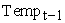to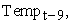and AP1 for each lowered decay factor is computed as input variables. The model was trained until the best result is found (model BM2). The model result shows that the r-value increases until it gets to its peak at k=0.75 and gradually decreases. This means that the decay factor of (k=0.75) gave the best result (Figure 5). It can be observed that the r-value (0.67) in model BM1 increased by 16% (to r=0.78) when the decay factor was lowered in model BM 2. The hydrograph of model BM2 also improved, compared to the wide range of underestimation of peak BM discharge observed in model BM1.

As the r values for the previous simulations were still low, the role of the potential evapotranspiration (ET) in the discharge was investigated. ET calculated using the Hargreaves method and Blaney-Criddle method were computed as additional input variables in model BM3 and model BM4, respectively. It was important to include ET as additional input variables to know how it influences BM discharge and to also improve the network model result. Model BM3 is computed with BM discharge as output variable while precipitation, API with best decay factor (k=0.75),and ET using the Hargreaves method is computed as input variables. The results show that ET using the Hargreaves method in model BM3 did not improve the result. This is evident in the model output with an r-value of 0.78, which is the same as model BM2 with the best decay factor. In model BM4, BM discharge is computed using the Blaney-Criddle equation to calculate ET. Consumptive-use crop coefficient (k) in the Blaney-Criddle ET equation depends solely on location, season, and vegetation type. Although many researchers like Xu and Singh 57 have mentioned measured k values for important crops at different locations, However, such measurements are hard to make and may also be subjected to errors due to the various diverse condition under which the studies were investigated 58. Not only does climate varies, but also soils, availability of water supply to crop, the method applied for consumptive use measurement, crop yield, and other factors varies from place to place. Thus, a variation in the k value can be expected. In addition, k values vary from 0.5 – 1.2 for orange trees and natural dense vegetation 51. Based on this, the k value used in this study for the Blaney-Criddle ET equation was adjusted and ANN is applied for each adjustment until the best result is found. It can be seen that the model run using a k value of 0.91 gave the highest r-value of 0.798, thereafter, the r-value sharply declines and remain steady afterward (Figure 6). Since ET using the Blaney Criddle method improved the result better than the Hargreaves method, it was selected for further model runs.

Since the r-value obtained for model BM4 is 0.798, there was a need to improve the result to get a higher r-value. To achieve this, more separated values of temperature up to 150 days were computed as additional input variables, and then the model is trained (model BM5). For this simulation, BM discharge is computed as output variable while API with best decay factor, precipitation, Blaney-Criddle ET with best k value (0.91), and separated values of temperature are computed as input variables. The result shows that as more temperature values are added as input variables, the r-value improves. The reason for this model improvement due to more temperature variables is because BM discharge has a component of baseflow, and air temperature has an impact on baseflow because it is the temperature of recharge in the groundwater system and then into the river. Although GMDH generates a mathematical formula for prediction, the formula generated in this simulation is too long due to the large number of input variables, making it inefficient and difficult to use.

• Figure 4. Model BM1 output using separated values of temperature (Tempt-1 to Tempt-9) and API with k = 0.95
• Figure 5. r values for all lowered decay factor
• Figure 6. r-value for each variation in k values of Blaney Criddle ET method

In the second approach to modeling BM (model BM6), the decay constant in both API and ATI is varied, and then trained until the best result is found (trial by error). An extraction from several trials by error model run based on the variation of decay factor in API and ATI shows that API with a decay factor of 0.73 and ATI with a decay factor of 0.90 recorded the best result, with an r-value greater than 0.74 (Figure 7). However, the r-value is lower than the best model in the first approach using separated values of temperature. Furthermore, the hydrograph shows a consistent underestimation of BM discharge across all time intervals.

• Figure 7. r values of the model run using variation in decay factor of API and ATI
• Figure 8. Model BM7 using GRNN, with the introduction of ATI

The third approach (model BM7) is to use input and output variables in the second approach but with a different ANN design architecture called GRNN. The model result shows an R squared and r-value of 0.81 and 0.90, respectively. Although only a few underestimations are observed in the hydrograph (Figure 8), it still shows a better match between the modeled and observed discharge, compared to the hydrograph in the second approach where a wide range of underestimation was observed. In general, the network using GRNN with the ATI method gives a more useful and efficient approach in predicting BM discharge. Although in GRNN, a mathematical formula is not produced unlike GMDH, it is possible to estimate or predict new discharges with the patterns established by the training and test sets and running the model again with the prediction set to determine unknown discharges.

RMSE, correlation coefficient (r), and R squared value were the performance measures that were selected for evaluation of all ANN models in BM. Of all the three approaches, model BM5 had the best performance based on the r-value and RMSE, however, the long mathematical formula generated from this simulation due to the large input variables has made it complicated to use in predicting BM discharge. Furthermore, the performance of models BM5 and BM7 was better than model BM6. For this paper, model BM 7 is preferred because of its reasonable and acceptable r value and RMSE, and its ease in predicting discharge by specifying a new discharge to be predicted in the training and test set pattern icon in the NeuroShell®2 program. The summary of performance for model BM7 is presented in Table 2. When the RMSE of the BM model is compared with the minimum and maximum range of values for the model output, the RMSE value for the model is acceptable.

6.2. Modeling of HF Flow

Modeling of BM discharge was conducted to identify the parameters influencing the discharge and to also be able to generate a prediction model using these parameters. Based on the overall model performance in BM, the input variables selected for calibrating flow in HF are API, BM discharge, precipitation, temperature, ATI, drainage area, and river mile. ANN using GMDH was applied for modeling of HF flow, with measured BM discharge, API, ATI, precipitation, temperature, HF drainage area, and HF river mile as input variables while HF discharge is computed as an output variable. The statistical performance of the model shows an r-value, R squared value, and RMSE of 0.97, 0.95, and 4.2cfs, respectively which indicates a good model output (Table 3). A plot of observed discharge (HF discharge) and modeled discharge (Figure 9) shows that the modeled discharge matches the actual peak discharge in HF010, HF060, and HF090 while an underestimation of discharge is observed from low discharge in HF090. Considering the values of the statistical performance of the model, it is seen from these model plots that the actual and modeled discharge have a reasonably good match. Based on the input variables, the model identifies all the input variables to be the most significant variables for predicting HF discharge. The network used all the input variables to generate the mathematical formula used in predicting HF discharge. Since there is continuous data for BM discharge, and precipitation, ATI, temperature, river mile, and drainage area of each HF site is known, the mathematical formula in Table 3 was used to simulate discharge for dates where discharge was not measured but chemical data is available, thereby creating ample data for modeling water chemistry in HF stream. To use this mathematical formula to predict HF discharge, the legends of the formula must be calculated, for instance in Table 3, (X1-X7) is calculated using the values of each input variable. These calculated values are then substituted in the mathematical formula to get the “Y” value. Since the Y value in the mathematical formula is known, it is then substituted for the “Y” value in the legend, to calculate HF discharge.

• Figure 9. Model for HF flow using GMDH
6.3. Modeling of Chemical Transport in HF Stream

ANN using GMDH is used to model chemical transport in HF. For each chemical concentration model, the plot of actual concentration and predicted concentration shows an underestimation in some HF sites, where the observed concentration reaches a peak faster than the modeled concentration, for instance, in the acidity concentration model, underestimation of acidity concentration is observed in HF039, HF095, and HF137 (Figure 10A) while in acidity load (Figure 10B), the model plot shows a good match between the modeled acidity load and observed acidity load in almost all HF sites. This similar match in the acidity model, where the load model displayed a better model match than the concentration model, is also observed with all other chemical models (Figure 10C-10F & Figure 11A-11F). However, the performance of all the models varies and is discussed in section 6.3.1.

Output from the ANN models for river chemistry in HF shows that, of all the input variables, HF discharge is the only variable that remains consistent as the most significant variable for all chemical concentration and load models, highlighting its influence in all the models. BM discharge is observed to only be less significant in the Mn concentration model while it is most significant for all other models. ATI is less significant in acidity load and alkalinity load but remains most significant in all other models. Furthermore, API is most significant in all models except for sulfate concentration and sulfate load where it is observed to be less significant.

• Figure 10. Model of chemical concentration/load for acidity, alkalinity, and Ca
• Figure 11. Model of chemical concentration/load for Mg, sulfate, and K

6.3.1. Model Performance for Chemical Concentration and Load Model in HF

Table 4 shows the summary of model performance for overall chemical concentration and load model in HF. The data for all chemical concentration models are indicated in red while the chemical load model is indicated in blue. For chemical concentration model, the r-value for Acidity, Al, Mn, Sulfate, Ca, Mg, K, Fe and Alkalinity are 0.78, 0.86, 0.87, 0.88, 0.87, 0.90, 0.88, 0.91, and 0.86, respectively, with highest r value (0.91) recorded in model for Fe concentration. RMSE for all the total datasets for chemical concentration range from 0.24 mg/l to 54.06 mg/l. Aluminum concentration with the lowest RMSE (0.24 mg/l) is identified as the model with the best performance. For the chemical load model, the r-value for all models was greater than 0.90, and the RMSE ranges from 4.60kg/day to 878.30kg/day. Mn load, with the lowest RMSE (4.60kg/day and r-value (0.96) demonstrates the best performance. The sulfate model had the highest RMSE in both the concentration model (54.06mg/l) and load model (878.30 kg/day), while a similar high RMSE trend also occurred in both Ca concentrations and load, and alkalinity concentration and load. When the RMSE of each chemical model is compared with the minimum and maximum range of values for the model output, the RMSE values for the models are acceptable.

Although the RMSE recorded in the overall model are acceptable, however, high RMSE values were still recorded in some chemical concentration models like sulfate concentration with RMSE of 54.06mg/l. Similarly, elevated values of RMSE were recorded for sulfate load (878 kg/day), calcium load (249.97 kg/day), and Alkalinity load (201.40 kg/day). To improve the model, it is important to have a lower RMSE because the lower the RMSE, the better the model prediction. To achieve this, it was necessary to examine the %error for each model run. A graph of %error vs HF discharge was plotted for each chemical concentration/load. HF discharge is selected because it is the only most significant variable that influences the prediction of both chemical concentration and load. Findings from the %error graph plot show that higher errors were dominated in HF discharge less than 12cfs while lower errors are dominated in discharge greater than 12cfs. Based on this evidence, the model was divided into two i.e (HF discharge less than 12cfs and HF discharge greater than 12cfs). The extraction was done in such a way that the entire data containing all input variables (HF discharge, BM discharge, Prep., API, temp, ATI, river mile, and drainage area) and output variables (chemical load and concentration) was organized from smallest to highest HF discharge and then separated into two data sets, one for discharge < 12cfs and the other for Hf discharge > 12cfs and ANN using GMDH was applied for each data set.

The statistical performance of the HF division model category for chemical concentration (Table 5) and load model (Table 6) shows the RMSE for each HF discharge division improved, especially in the HF discharge >12cfs category for chemical concentration model and in HF discharge <12cfs category for chemical load model, compared to when the model was performed without division. In general, based on the performance of all these models, the input variables have a good capacity to predict chemical concentration/load in the HF stream.

### 7. Conclusions

The study aimed to investigate the potential of ANN models for simulating the hydrologic behavior in the Raccoon Creek watershed (with a BM gaged station) and subsequently use the model parameters for BM to predict flow and chemistry of Hewett Fork subwatershed, a tributary of raccoon creek watershed, southeast Ohio. In this regard, we set up an ANN model (using NeuroShell®2) to generate streamflow prediction of the watershed. One major challenge in the ANN model for BM is the consistent underestimation of discharge, where the network is unable to match modeled discharge with observed peak discharge, hence, the reason why BM flow is modeled in three approaches. The disadvantage of the GMDH model in the first approach is the long mathematical formula generated due to the large input variable considered, making it difficult to predict BM flow. However, the introduction of ATI in the GRNN model is considered more efficient due to its performance compared to GMDH, where a wide range of underestimation of discharge is observed. Also, the results obtained with the GRNN model in the third approach are better than GMDH models. In general, findings from the modeling of BM flow show that API has a strong influence on the prediction of BM flow. This is evident in the model improvement observed after the decay factor for API was decreased. The lower value for the decay factor (0.73) for API can be understood if we consider the geology of the area. The soils are rich in clays and that makes the overland flow greater and the decay of the effect of precipitation can last longer due to more water reaching the stream from the far regions of the watershed. Furthermore, air temperature also plays a major role in BM flow prediction. This is because BM discharge has a component of baseflow, and air temperature has an impact on baseflow and overland flow because it is the temperature of recharge in the groundwater system and then into the river. The GRNN model has proven more effective in modeling discharge at BM because it gives a high r-value (0.90) without the complexity of a very large equation. Prediction input data can be added to the model to get the expected discharge. The GMDH model for the prediction of HF flow using the model parameter for BM flow shows a good model output with an r-value of 0.97 and an RMSE of 4.18cfs. It should be noted that HF discharge is not measured often and this modeling approach for the discharge helps to create more flow data for modeling of water chemistry. Since a continuous flow record is not documented for HF flow, this mathematical formula will be useful for water resource practitioners and researchers to create a continuous flow estimate for HF flow (an ungaged AMD stream). The result of the water chemistry model using GMDH, with r values greater than 0.80 for each model, shows that the input variables have a good capacity to predict chemical concentration/load in the HF stream. It was observed that HF discharge is the only variable that remains consistent as the most significant variable for all chemical concentration and load models, highlighting its influence in all the models. Furthermore, when the overall chemical concentration and load model was divided based on HF discharge, the statistical performance of the HF sub-models for chemical concentration and load model shows the RMSE for each HF discharge sub-model improved, especially in the HF discharge >12cfs category for chemical concentration model and in HF discharge <12cfs category for chemical load model, compared to when the model was performed without division. Based on the findings established in this study, it is recommended that similar modeling work should be conducted on other river basins in this region and the world if appropriate data is available. Furthermore, the study should involve incorporating additional river basin characteristics such as morphology, slope, surface roughness features, etc, as input variables to generate a more robust prediction of streamflow.

### Acknowledgments

The authors would like to thank Dr. Ryan Fogt of Ohio University, US, for providing the meteorological datasets in the Scalia Laboratory. we would want to thank Dr. Natalie Kruse, Dr. Alycia Stigall, and Dr. Gregory Springer for their valuable suggestions and comments that helped improve the quality of this paper. Also, we would like to thank Department of Geosciences, Baylor University, USA, for providing funds for the publication fee.

### References

Published with license by Science and Education Publishing, Copyright © 2021 Toluwaleke Ajayi, Dina L.Lopez and Abiodun E.Ayo-BaliThis work is licensed under a Creative Commons Attribution 4.0 International License. To view a copy of this license, visit http://creativecommons.org/licenses/by/4.0/